SSC  >  Logical Reasoning - Test 1

# Logical Reasoning - Test 1

Test Description

## 25 Questions MCQ Test SSC CGL Tier 1 Mock Test Series | Logical Reasoning - Test 1

Logical Reasoning - Test 1 for SSC 2022 is part of SSC CGL Tier 1 Mock Test Series preparation. The Logical Reasoning - Test 1 questions and answers have been prepared according to the SSC exam syllabus.The Logical Reasoning - Test 1 MCQs are made for SSC 2022 Exam. Find important definitions, questions, notes, meanings, examples, exercises, MCQs and online tests for Logical Reasoning - Test 1 below.
Solutions of Logical Reasoning - Test 1 questions in English are available as part of our SSC CGL Tier 1 Mock Test Series for SSC & Logical Reasoning - Test 1 solutions in Hindi for SSC CGL Tier 1 Mock Test Series course. Download more important topics, notes, lectures and mock test series for SSC Exam by signing up for free. Attempt Logical Reasoning - Test 1 | 25 questions in 15 minutes | Mock test for SSC preparation | Free important questions MCQ to study SSC CGL Tier 1 Mock Test Series for SSC Exam | Download free PDF with solutions
 1 Crore+ students have signed up on EduRev. Have you?
Logical Reasoning - Test 1 - Question 1

### Select the related word from the given alternatives. BIRD : AEROPLANE : : FISH : ?

Detailed Solution for Logical Reasoning - Test 1 - Question 1

A bird’s mode of transport is through the air. The man-made creation that serves a similar purpose is the aeroplane.
Similarly, a fish’s medium of transport is through water. The corresponding man-made mode of transport is a boat.

Logical Reasoning - Test 1 - Question 2

### Select the related letters from the given alternatives. DCBA : WXYZ : : IJKL : ?

Detailed Solution for Logical Reasoning - Test 1 - Question 2

The first phrase consists of letters written in reverse alphabetical order (D → C → B → A). The corresponding phrase is in regular alphabetical order (W → X → Y → Z).
Similarly, the given phrase is in regular alphabetical order (I → J → K → L). Therefore, the required word must be in reverse alphabetical order.
Further, the last letter of the first phrase (A) is the first letter of the alphabet, and the last of the second word (Z) is the first in reverse alphabetical order.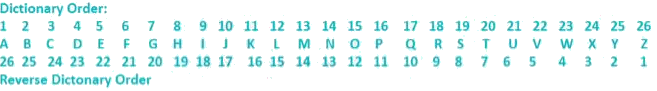L is the 12th in regular order, and therefore the last letter of the required word must end with the same position in reverse order, i.e. O.
Therefore, the required word is RQPO.

Logical Reasoning - Test 1 - Question 3

### Select the related numbers from the given alternatives. 381 : 160 : : 478 : ?

Detailed Solution for Logical Reasoning - Test 1 - Question 3

The difference between the first pair is 221, i.e. 381 – 160 = 221.
Therefore, the required answer will be 478 – 221 = 257.

Logical Reasoning - Test 1 - Question 4

Find the odd word from the given alternatives.

Detailed Solution for Logical Reasoning - Test 1 - Question 4

Affluent, Rich and Well-to-do all refer to high level of material wealth. However, prestigious refers more to stature or achievements than material wealth.

Logical Reasoning - Test 1 - Question 5

Find the odd letters from the given alternatives.

Detailed Solution for Logical Reasoning - Test 1 - Question 5

By rearranging the letters such that the second and first place are interchanged, the letters should be every alternate letter in the alphabet.
1) OMQ → MOQ → M N O P Q
2) TPR → PTR → P Q R S T → Does not satisfy.
3) TRV → RTV → R S T U V
4) VTX → TVX → T U V W X
Therefore, TPR is the odd answer.

Logical Reasoning - Test 1 - Question 6

Find the odd number pair from the given alternatives.

Detailed Solution for Logical Reasoning - Test 1 - Question 6

There is a difference of three between each pair.
11 – 8 = 3, 4 – 1 = 3, 10 – 7 = 3.
However, 5 – 3 = 2, and therefore this is the odd one out

Logical Reasoning - Test 1 - Question 7

Arrange the following words as per order in the dictionary.
1. Launch, 2. Laugh, 3. Lattice, 4. Latent, 5. Latitude

Detailed Solution for Logical Reasoning - Test 1 - Question 7

Words in dictionary order are listed such that they are in alphabetical order on the basis of the first letter they differ in. Therefore, the order will be :
Latent (4), Latitude (5), Lattice (3), Laugh (2), Launch (1).

Logical Reasoning - Test 1 - Question 8

A series is given, with one term missing. Choose the correct alternative from the given ones that will complete the series.

XFB, UGD, RIG, OLK, ?

Detailed Solution for Logical Reasoning - Test 1 - Question 8

The relation between the letters is as follows,
X – 3 = U, U – 3 = R, R – 3 = O, O – 3 = L
F + 1 = G, G + 2 = I, I + 3 = L, L + 4 = P
B + 2 = D, D + 3 = G, G + 4 = K, K + 5 = P
Hence the next term is LPP.

Logical Reasoning - Test 1 - Question 9

A series is given, with one term missing. Choose the correct alternative from the given ones that will complete the series.

1, 2, 4, 7, ?, 16

Detailed Solution for Logical Reasoning - Test 1 - Question 9

The difference between each increases by one.
2 – 1 = 1
4 – 2 = 2
7 – 4 = 3
Therefore, the required number will be 7 + 4 = 11, or 16 – 5 = 11.

Logical Reasoning - Test 1 - Question 10

A & B are married couple. X and Y are brothers. A is the brother of X. How is Y related to B?

Detailed Solution for Logical Reasoning - Test 1 - Question 10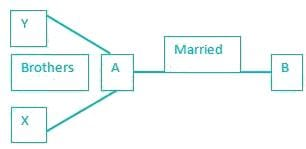therefore, since X and Y are brothers, and X and A are brothers, Y and A are brothers. This makes Y the brother in law of B.

Logical Reasoning - Test 1 - Question 11

A is shorter than B but taller than C. D is shorter than A but taller than C and E is shorter than B but taller than A. The shortest person is ?

Detailed Solution for Logical Reasoning - Test 1 - Question 11

1) A is shorter than B but taller than C.
⇒ C < A < B
2) D is shorter than A but taller than C.
⇒ C < D < A < B
3) E is shorter than B but taller than A.
⇒ C < D < A < E < B
Therefore, C is the shortest.

Logical Reasoning - Test 1 - Question 12

From the given words, select the word which cannot be formed using the letters of the given word.

DISSERTATION

Detailed Solution for Logical Reasoning - Test 1 - Question 12

Let us consider each option.
1) DISSERTATION → SISTER
2) DISSERTATION → STATION
3) DISSERTATION → DIRECTION
4) DISSERTATION → RATION.
There is no ‘C’ in dissertation, and therefore, direction cannot be formed.

Logical Reasoning - Test 1 - Question 13

If A = 1, E = 5, then HEAR = ?

Detailed Solution for Logical Reasoning - Test 1 - Question 13

Each letter is denoted by its corresponding numeric place in the alphabet.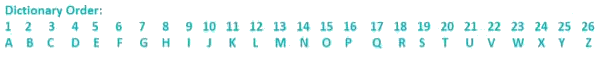Therefore, H = 8, E = 5, A = 1 and R = 18.
On adding these, we get 8 + 5 + 1 + 18 = 32.

Logical Reasoning - Test 1 - Question 14

If P denotes +, Q denotes - , R denotes '÷', and S denotes ×, then : 18S36R12Q6P7 = ?

Detailed Solution for Logical Reasoning - Test 1 - Question 14

By putting in the required operators into the system, we get :
18 × 36 / 12 – 6 + 7
On applying BODMAS, we get
18 x (36/12) – 6 + 7
= (18 x 3) – 6 + 7
= 54 + 1
= 55

Logical Reasoning - Test 1 - Question 15

Some equations have been solved on the basis of certain system. Find the answer for the unsolved equation on that basis. If

98 - 39 - 27 = 31,
87 - 38 - 34 = 20,
then 79 - 25 - 12 = ?

Detailed Solution for Logical Reasoning - Test 1 - Question 15

Here the logic is as follows,
9 × 8 = 72, 3 × 9 = 27, 2 × 7 = 14
⇒ 72 – 27 – 14 = 31
8 × 7 = 56, 3 × 8 = 24, 3 × 4 = 12
⇒ 56 – 24 – 12 = 20
Similarly,
7 × 9 = 63, 2 × 5 = 10, 1 × 2 = 2
⇒ 63 – 10 – 2 = 51
Hence the required number is 51.

Logical Reasoning - Test 1 - Question 16

Select the missing number from the given responses :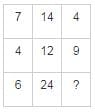Detailed Solution for Logical Reasoning - Test 1 - Question 16

Here the relation is as follows : column 1 × √column 3 = column 2
Thus,
7 × √4 = 7 × 2 = 14
4 × √9 = 4 × 3 = 12
Similarly,
6 × √x = 24
√x = 24/6
x = 42
x = 16
Hence the required number is 16.

Logical Reasoning - Test 1 - Question 17

Rahul walks 30 meters towards south. Then turns to his right and starts walking straight till he completes another 30 meters. Then again turning to his left he walks for 20 meters. He then turns to his left and walks for 30 meters. How far is he from his initial position?

Detailed Solution for Logical Reasoning - Test 1 - Question 17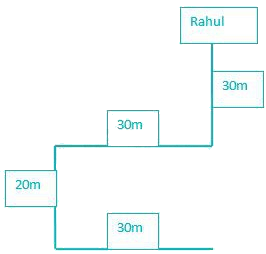Since Rahul has walked 30 meters to the right, and later 30 meters to the left, he is back in the same line which he originally started in.
Therefore, the total distance from the initial point will be given by the distance he covered towards the south, i.e. 30 + 20 = 50 meters.

Logical Reasoning - Test 1 - Question 18

You have to consider the statement to be true, even if it seems to be at variance from commonly known facts. You have to decide which of the given conclusions, if any, follow from the given statements.
Statements:
All teachers are actors.
Some actors are women.
Conclusion:
I. All teachers are women.
II. Some women are teachers.
III. Some women are actors.
IV. All actors are teachers.

Detailed Solution for Logical Reasoning - Test 1 - Question 18

each statement after analyzing the least possible Venn diagram.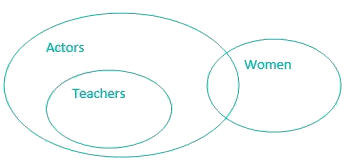Conclusions:
I. All teachers are women → It’s possible but not definite, hence false.
II. Some women are teachers → It’s possible but not definite, hence false.
III. Some women are actors → It’s definite, hence true.
IV. All actors are teachers → It’s possible but not definite, hence false.
Therefore, only conclusion III will follow.

Logical Reasoning - Test 1 - Question 19

How many triangles are there in the following figure PQRS?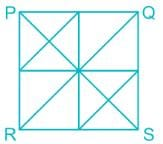Detailed Solution for Logical Reasoning - Test 1 - Question 19

Let us check the number of triangles formed,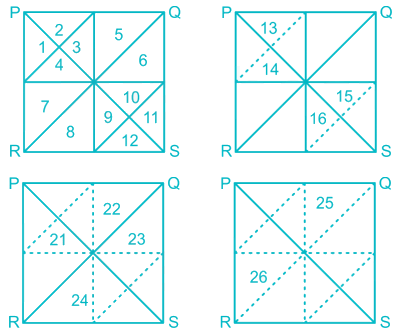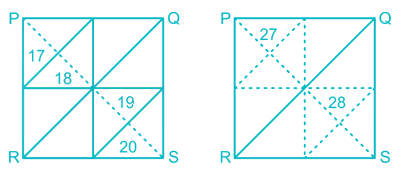Hence 28 triangles are formed.

Logical Reasoning - Test 1 - Question 20

Identify the diagram that best represents the relationship among classes given below : Stationery items, Pencils, Potatoes

Detailed Solution for Logical Reasoning - Test 1 - Question 20

As we all know Pencil comes under Stationery items whereas Potatoes is an edible item.

So the correct Venn diagram is as follows,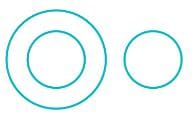Logical Reasoning - Test 1 - Question 21

Which answer figure will complete the pattern in the question figure?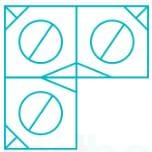Detailed Solution for Logical Reasoning - Test 1 - Question 21

The required image should have circle in middle with a diameter from north - east to south - west. There should be a slanting line at upper left corner and a ‘T’ at lower right corner.

Hence the required image is as shown,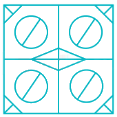Logical Reasoning - Test 1 - Question 22

From the given answer figures, select the one in which the question figure is hidden/embedded.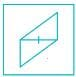Detailed Solution for Logical Reasoning - Test 1 - Question 22

The image given in question is hidden in the following image as shown,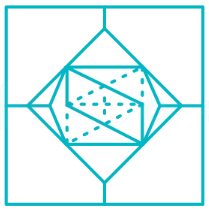Logical Reasoning - Test 1 - Question 23

A piece of paper is folded and cut as shown below in the question figures. From the given answer figures, indicate how it will appear when opened.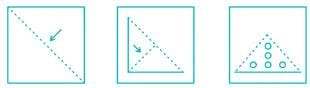Detailed Solution for Logical Reasoning - Test 1 - Question 23

When we open the paper step by step, we get the following figure.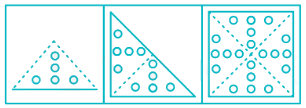Logical Reasoning - Test 1 - Question 24

If a mirror is placed on the line MN, then which of the answer figures is the right image of the given figure?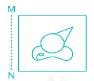Detailed Solution for Logical Reasoning - Test 1 - Question 24

The mirror image of the given figure is as shown,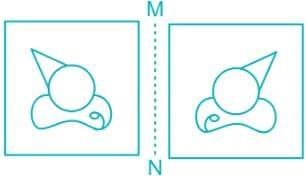Logical Reasoning - Test 1 - Question 25

In this question, the sets of numbers given in the alternatives are represented. The columns and rows of Matrix I are numbered from 0 to 4 and that of Matrix II are numbered from 5 to 9. A letter from these matrices can be represented first by its row and next by its column, e.g., A can be represented by 02, 14, etc., and 'O' can be represented by 55, 67, etc. Similarly you have to identify the set for the word 'LEND'.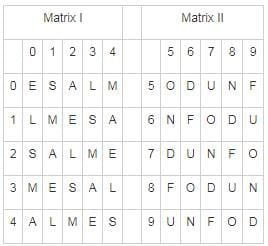Detailed Solution for Logical Reasoning - Test 1 - Question 25

Let’s check each option,
1) 34, 43, 40, 56 → LEAD
2) 10, 31, 77, 99 → LEND
3) 41, 12, 41, 59 → LELF
4) 22, 14, 65, 75 → LAND
Hence the correct representation of the word LEND is 10, 31, 77, 99.

## SSC CGL Tier 1 Mock Test Series

42 docs|103 tests
 Use Code STAYHOME200 and get INR 200 additional OFF Use Coupon Code
Information about Logical Reasoning - Test 1 Page
In this test you can find the Exam questions for Logical Reasoning - Test 1 solved & explained in the simplest way possible. Besides giving Questions and answers for Logical Reasoning - Test 1, EduRev gives you an ample number of Online tests for practice

## SSC CGL Tier 1 Mock Test Series

42 docs|103 tests